ICSE Class 7CISCE
Share

# Selina solutions for Class 7 Chemistry chapter 7 - Air and Atmosphere

#### Selina Selina Concise Chemistry - Middle School Class 7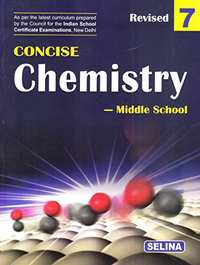## Chapter 7: Air and Atmosphere

#### Selina solutions for Class 7 Chemistry Chapter 7 [Page 0]

Give one use for  the following inert gases :

argon

Give one use for the following inert gase :

helium

Give one use for the following inert gase :

neon

Give one use for the following inert gase :

Give one use for  the following inert gase :

krypton

Give one use for the following inert gase :

xenon

Answer the question put against the following constituents of air:

Nitrogen : Explain its significance for plants and animals.

Answer the question put against the following constituents of air :

Oxygen : What is the percentage proportion of oxygen in air ? Why is oxygen called active air.

Answer the question put against the following constituents of air :

Carbon dioxide : “Although carbon dioxide plays no role in respiration, all life would come to an end if there is no carbon dioxide in air.” Support this statement with relevant facts.

Answer the question put against the following constituents of air :

Water vapours : Explain their role in modifying the earth’s climate.

Define the term pollutants.

Define the term acid rain.

Define the term Global warming.

Define the term smog.

“Air is a mixture”. Support this statement citing at least three evidences.

What is air pollution ? What are the harmful effects of sulphur dioxide, nitrogen dioxide and hydrogen sulphide present in the air ?

What are the causes of air pollution ?

Suggest five measures to prevent air pollution.

What is nitrogen-fixation?

What are the two ways in which nitrogen fixation occurs?

Explain the conversion of nitrogen into nitrates during lightning.

#### Selina solutions for Class 7 Chemistry Chapter 7 [Page 0]

The most abundant element in the earth’s crust.

A chemical called oxygenated water.

A metal highly resistant to rusting.

A mixture of oxygen and carbon dioxide used for artificial respiration.

Two substances from which oxygen can be obtained at a large scale.

An oxide and a carbonate containing oxygen.

Two substances which undergo rapid oxidation.

Taking hydrogen peroxide, how would you prepare oxygen in the laboratory ?

What is the role of manganese dioxide in the preparation of oxygen ?

Write the balanced chemical equation for the above chemical reaction.

Why is hydrogen peroxide preferred in the preparation of oxygen gas ?

Why is oxygen collected by downward displacement of water ?

What happens when a glowing splinter is introduced in a jar containing oxygen ?

What happens when oxygen gas is passed through alkaline pyrogallol solution ?

What happens when mercuric oxide is heated?

What happens when potassium nitrate is heated

Why is potassium chlorate not used for laboratory preparation of oxygen ?

What are oxides ?

Give two examples of metallic oxides.

Give two examples of non-metallic oxides.

Name the three types of oxidation processes. In which of these large amount of heat and light energy are produced?

What do you observe when Sulphur is heated and then tested with moist blue and red litmus – paper?

What do you observe when Phosphorus is heated and then tested with moist blue and red litmus – paper?

What do you observe when Calcium is heated and then tested with moist blue and red litmus – paper?

What do you observe when Magnesium is heated and then tested with moist blue and red litmus – paper?

Complete and balance the following chemical equation.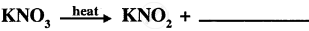Complete and balance the following chemical equation.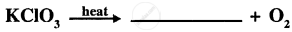Complete and balance the following chemical equation.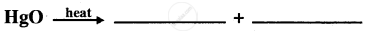Complete and balance the following chemical equation.

CaO + H2O → ____________

Give four uses of oxygen.

How is oxygen naturally renewed in air ?

What is rust ?

State at least two ways of prevent rusting.

State two differences between : Rusting and burning.

#### Selina solutions for Class 7 Chemistry Chapter 7 [Page 0]

Fill in the blank :

________ is the most abundant inert gas present in air.

Fill in the blanks :

Oxides of sulphur and nitrogen combine with rain water to form ___________ and _________ which cause ____________.

Fill in the blanks :

_______ and ______ are the most common air pollutants.

Fill in the blank :

____________ discovered the oxygen gas.

Fill in the blank :

Oxygen occupies about _____% of air by volume.

Match the following :

 Column A Column B (a) Global warming 1. Hydrated ferric oxide (b) Acid rain 2. Manganese dioxide (c) Rust 3. Carbon dioxide (d) Catalyst 4. Methane (e) Photosynthesis 5. Nitrogen Dioxide

MULTIPLE CHOICE QUESTIONS:

A fuel when used releases least amount of pollutants in the air.
(a) sulphur dioxide
(b) chlorofluorocarbon
(c) smoke
(d) CNG

MULTIPLE CHOICE QUESTION:
The natural way of adding oxygen to air which involves green plants is called
(a) photosynthesis
(b) respiration
(c) burning
(d) dissolution

MULTIPLE CHOICE QUESTION:

Which one of the following is most likely to be corroded?
(a) a stainless steel cup-board
(b) a galvanised iron bucket
(c) an iron hammer
(d) a tin plated iron box

MULTIPLE CHOICE QUESTION:

The process by which oxidation of food in our body takes place is
(a) photosynthesis
(b) respiration
(c) decomposition
(d) combustion

#### Selina solutions for Class 7 Chemistry Chapter 7 [Page 0]

What was the ancient belief regarding the nature of matter ?

Name the main components of air.

Mention the contribution of the following scientists :

1. Antoine Lavoisier
2. Robert Boyle
3. Joseph Priestley
4. Sir W. Ramsay

State three uses of water vapour in the air.

State one method by which :

1. Oxygen is added to air
2. Carbon dioxide is added to air
3. Oxygen is removed from the air
4. Carbon dioxide is removed from the air

Describe a simple experiment to show that l/5th of air is oxygen.

Give reasons to support the fact that air is a mixture.

Name the products formed when a candle burns in air.

#### Selina solutions for Class 7 Chemistry Chapter 7 [Page 0]

Fill in the blank by choosing the correct word from the given list.

List: oxygen, carbonic, suIphur dioxide gas, precipitation, helium

____________ gas can cause acid rain.

Fill in the blank space by choosing the correct word from the given list.

List: oxygen, carbonic, suIphur dioxide gas, precipitation, helium

Every nine parts of water by weight contains eight parts by weight of _________.

Fill in the blank space by choosing the correct word from the given list.
List: oxygen, carbonic, suIphurUioxide gas, precipitation, helium

Dust particles help in the ________ of water vapour in air.

Fill in the blank space by choosing the correct word from the given list.
List: oxygen, carbonic, suIphurUioxide gas, precipitation, helium

_______ gas is used for filling weather observation balloons.

Fill in the blank space by choosing the correct word from the given list.
List: oxygen, carbonic, suIphurUioxide gas, precipitation, helium

Solution of carbon dioxide in water is called _________ acid.

Statement given below is incorrect. Write the correct statement :

Carbon dioxide gas allows the earth to radiate out heat rapidly.

Statement given below is incorrect. Write the correct statement :

Helium gas at low pressure is used for filling electric bulbs.

Statement given below is incorrect. Write the correct statement :

Symbiotic bacteria are present in nodules of cereal plants such as wheat.

Statement given below is incorrect. Write the correct statement :

During thunder and lightning, nitrogen and oxygen combine to form nitrogen dioxide gas.

Statement given below is incorrect. Write the correct statement :

The percentage of oxygen in air by volume, varies from 21% to 22%.

Match the statements in Column A, with those in Column B.

 Column A Column B 1. Largest Constituent of air, which dilutes the activity of oxygen. (a) Oxygen 2. A gas used for filling weather observeation balloons. (b) Carbonic acid 3. An acid formed by the dissolution of sulphur dioxide gas in water. (c) Sulphuric acid 4. An acid formed by the dissolution of carbon dioxide in water. (d) Helium 5. A gas vital for respiration. (e) Nitrogen

Write ‘True’ or ‘False’ in front of following statement.

Atmospheric nitrogen is directly absorbed by the plants to form plant proteins.

Write ‘True’ or ‘False’ in front of following statement.

Water containing dissolved carbon dioxide can dissolve marble.

Write ‘True’ or ‘False’ in front of following statement.

Nitrogen is a gaseous non-metal essential for respiration.

Write ‘True’ or ‘False’ in front of following statement.

The area where a lot of fuel burns has more percentage of carbon dioxide.

Write ‘True’ or ‘False’ in front of following statement.

The trapping of solar heat energy in the earth’s atmosphere is called greenhouse effect.

Tick (√) the most appropriate answer.

A gas which dilutes the activity of oxygen in air is :
(a) carbon dioxide
(b) sulphur dioxide
(c) nitrogen
(d) noble gases

Tick (√) the most appropriate answer.

Symbiotic bacteria is not present in the nodules of :
(a) gram plant
(b) rice plant
(c) sweet peas plant
(d) peas plant

Tick (√) the most appropriate answer.

The presence of water vapour in air is essential for :
(a) health and comfort of animals
(b) rate of evaporation from plants and animals
(c) growth of plants
(d) all the above

Tick (√) the most appropriate answer.

Which is not a polluting gas for the air?
(a) Sulphur dioxide gas
(b) Nitrogen dioxide gas
(c) Carbon monoxide gas
(d) Neon gas

Tick (√) the most appropriate answer.

The humans pollute the air by :
(a) burning a vast amount of coal
(b) burning waste materials
(c) using petrol and diesel vehicles
(d) all the above

Briefly state the importance of Oxygen.

Briefly state the importance of Nitrogen.

Briefly state the importance of Carbon dioxide.

Briefly state the importance of Water vapour.

What do you understand by the term polluted air?

State and explain two ways by which air gets polluted in nature.

Name and briefly explain four ways by which pollution of air is caused by humans.

Name and briefly explain four ways by which pollution of air caused by humans is minimised.

What is greenhouse effect?

How is greenhouse effect produced?

Discuss harmful effects of greenhouse effect.

What is the significance of water vapour in air?

Name three noble gases present in the air. State their one industrial use

What problems are caused by the presence of dust particles in excessive amount in the air?

What problems are caused by the presence of smoke particles in excessive amount in the air?

What problems are caused by the presence of sulphur dioxide gas in excessive amount in the air?

What problems are caused by the presence of  nitrogen dioxide gas in excessive amount in the air?

What problems are caused by the presence of carbon monoxide gas in excessive amount in the air?

What problems are caused by the presence of hydrorgen sulphide in excessive amount in the air?

#### Selina solutions for Class 7 Chemistry Chapter 7 [Page 0]

Fill in the blank:

_____________ discovered the oxygen gas.

Fill in the blank:

Oxygen is slightly _________ than air.

Fill in the blank:

Manganese dioxide acts as a _________.

Fill in the blanks:

Oxygen is collected by the __________ displacement of ________.

Fill in the blank:

Oxygen occupies about ______ of air by volume.

Fill in the blank:

Oxygen is ___________ to litmus testing.

What happens when mercuric oxide is heated ?

What happens when potassium nitrate is heated ?

Why is potassium chlorate is not used for laboratory preparation of oxygen ?

What happens when Sulphur burns in oxygen ? Also give the balanced chemical equation for the reaction.

What happens when Carbon burns in oxygen ? Also give the balanced chemical equation for the reaction.

What happens when Phosphorus burns in oxygen ? Also give the balanced chemical equation for the reaction.

What happens when Sodium burns in oxygen ? Also give the balanced chemical equation for the reaction.

What happens when Magnesium burns in oxygen ? Also give the balanced chemical equation for the reaction.

What happens when Iron burns in oxygen ? Also give the balanced chemical equation for the reaction.

Give balanced equation to obtain oxygen from Red lead.

Give balanced equation to obtain oxygen from Silver oxide.

Give balanced equation to obtain oxygen from Potassium chlorate.

Give balanced equation to obtain oxygen from Hydrogen peroxide.

Give balanced equation to obtain oxygen from Mercuric oxide.

Give balanced equation to obtain oxygen from Lead dioxide.

State the use of manganese dioxide in the laboratory prepa-ration of oxygen from potassium chlorate.

State the precautions while preparing oxygen in the labo-ratory.

How will you obtain dry oxygen in the laboratory ?

How will you identify oxygen in the laboratory ?

Classify Water. Also give a balanced equation to prepare it.

Classify Carbon dioxide. Also give a balanced equation to prepare it.

Classify Calcium oxide. Also give a balanced equation to prepare it.

Classify Aluminium oxide. Also give a balanced equation to prepare it.

Classify Copper oxide. Also give a balanced equation to prepare it.

Classify Carbon monoxide. Also give a balanced equation to prepare it.

Classify Sulphur dioxide. Also give a balanced equation to prepare it.

Classify Phosphorus pentoxide. Also give a balanced equation to prepare it.

Classify Nitric oxide. Also give a balanced equation to prepare it.

Classify Magnesium oxide. Also give a balanced equation to prepare it.

State two characteristics of Acidic oxides.

State two characteristics of Basic oxides.

Complete and balance the following equation

P2O5   +  H2O   →

Complete and balance the following equation

S   +   O2    →

Complete and balance the following equation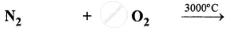Complete and balance the following equation

Al   +  O2   →

Complete and balance the following equation

K2O    +    H2O   →

Complete and balance the following equation

CaO    +    H2O    →

#### Selina solutions for Class 7 Chemistry Chapter 7 [Page 0]

Fill in the blank space by choosing the correct word from the given list.

List: sulphurous, nitric acid, red lead oxide paint, oxygen, phosphoric

Potassium nitrate crystals on heating strongly yield potassium nitrite and ________ gas.

Fill in the blank space by choosing the correct word from the given list.

List: sulphurous, nitric acid, red lead oxide paint, oxygen, phosphoric

Sulphur dioxide gas dissolves in water to form ___________ acid.

Fill in the blank space by choosing the correct word from the given list.

List: sulphurous, nitric acid, red lead oxide paint, oxygen, phosphoric

Phosphorus pentaoxide on dissolving in water forms _________ acid.

Fill in the blank space by choosing the correct word from the given list.
List: sulphurous, nitric acid, red lead oxide paint, oxygen, phosphoric

To prevent rusting, the underside of the ships is coated with __________________.

Fill in the blank space by choosing the correct word from the given list.
List: sulphurous, nitric acid, red lead oxide paint, oxygen, phosphoric

The nitric oxide gas reacts with the oxygen and water vapour to form ______ vapour.

Statement given below is incorrect. Write the correct statement :

Oxygen is prepared in laboratory by treating hydrogen oxide with manganese dioxide.

Statement given below is incorrect. Write the correct statement :

Calcium burns in oxygen with a golden yellow flame.

Statement given below is incorrect. Write the correct statement :

The oxygen gas turns moist blue litmus red.

Statement given below is incorrect. Write the correct statement :

Moist oxygen causes rusting in carbon.

Statement given below is incorrect. Write the correct statement :

Potassium nitrate on strong heating decomposes into potassium nitrite and nitrogen gas.

Match the statements in Column A, with those in Column B.

 Column A Column B 1. Largest Constituent of air, which dilutes the activity of oxygen. (a) Oxygen 2. A gas used for filling weather observation balloons. (b) carbon acid 3. An acid formed by the dissolution of sulphur dioxide gas in water. (c) Sulphur acid 4. An acid formed by the dissolution of carbon dioxide in water. (d) Helium 5. A gas vital for respiration. (e) Nitrogen

Write ‘True’ or ‘False’ in front of following statement.

The catalyst used in laboratory preparation of oxygen gas from hydrogen peroxide gas is magnesium oxide

Write ‘True’ or ‘False’ in front of following statement.

Under laboratory conditions, the oxygen gas is insoluble in water.

Write ‘True’ or ‘False’ in front of following statement.

The rust formed on the surface of iron easily crumbles.

Write ‘True’ or ‘False’ in front of following statement.

Enamelling is the process of baking a mixture of silicates on the surface of aluminium at a high temperature.

Write ‘True’ or ‘False’ in front of following statement.

Liquid oxygen is used for burning fuel in spaceships.

Tick (√ ) the most appropriate answer.

The catalyst used in the preparation of oxygen from hydrogen peroxide is :
(a) Magnesium oxide
(b) Manganese oxide
(c) Manganese dioxide
(d) Manganese hydroxide

Tick (√ ) the most appropriate answer.

Sodium burns in oxygen with a flame which is :
(a) brick red in colour
(b) golden yellow in colour
(c) blue in colour
(d) crimson in colour

Tick (√ ) the most appropriate answer.

Galvanising is the process of coating iron with a layer of molten :
(a) zinc
(b) tin
(c) copper
(d) silver

Tick (√ ) the most appropriate answer.

Magnesium burns with a dazzling white flame to form magnesium oxide, a powdery mass. The colour of magnesium oxide is : .
(a) milk white
(b) grey
(c) silvery
(d) yellow

Tick (√ ) the most appropriate answer.

When sulphur burns in oxygen, the colour of the flame is :
(a) dazzling white
(b) golden yellow
(c) parrot green
(d) brilliant blue

Describe your observations when potassium nitrate crystals are heated strongly in a test tube and a glowing splint is held near the mouth of test tube.

Write a fully balanced chemical equation for the decomposition of potassium nitrate crystals.

(a) Starting from hydrogen peroxide how is oxygen gas prepared in laboratory?
(b) What is the function of manganese dioxide?
(c) Write fully balanced equation for the liberation of oxygen from hydrogen peroxide.
(d) Why is the above method preferred to any other method?

State any four physical properties of oxygen gas.

Describe what you will observe and write fully balanced equation when the following is introduced in a jar of oxygen.

Red hot coal (carbon)

Describe what you will observe and write fully balanced equation when the following is introduced in a jar of oxygen.

Burning sulphur

Describe what you will observe and write fully balanced equation when the following is introduced in a jar of oxygen.

Burning sodium

Describe what you will observe and write fully balanced equation when the following is introduced in a jar of oxygen.

Burning magnesium

Describe what you will observe and write fully balanced equation when the following is introduced in a jar of oxygen.

Burning phosphorus

How will you prove that oxide formed by burning sodium is basic in nature.

How will you prove that oxide formed by sulphur is acidic in nature?

Write fully balanced equation for the following :

CO2  +  H2O  →

Write fully balanced equation for the following :

Ca(OH)2  +  CO2   →

Write fully balanced equation for the following :

SO2   +   H2O  →

Write fully balanced equation for the following :

P2O5    +   H2O   →

Write fully balanced equation for the following :

Na2O   +   H2O   →

Write fully balanced equation for the following :

MgO   +   H2O   →

State three uses of oxygen, other than artificial respiration.

Give tests for oxygen gas.

What is rust ?

What is rusting?

State two most important conditions for rusting.

State four ways of preventing rusting.

## Chapter 7: Air and Atmosphere

#### Selina Selina Concise Chemistry - Middle School Class 7## Selina solutions for Class 7 Chemistry chapter 7 - Air and Atmosphere

Selina solutions for Class 7 Chemistry chapter 7 (Air and Atmosphere) include all questions with solution and detail explanation. This will clear students doubts about any question and improve application skills while preparing for board exams. The detailed, step-by-step solutions will help you understand the concepts better and clear your confusions, if any. Shaalaa.com has the CISCE Selina Concise Chemistry - Middle School for Class 7 solutions in a manner that help students grasp basic concepts better and faster.

Further, we at Shaalaa.com provide such solutions so that students can prepare for written exams. Selina textbook solutions can be a core help for self-study and acts as a perfect self-help guidance for students.

Concepts covered in Class 7 Chemistry chapter 7 Air and Atmosphere are Concept of Air - Mixture of Gases., Composition of Air and Uses of Its Components., Concept for Oxygen - Combustion., Mass Change During Burning (Burning of Magnesium and Candle)., Equations for Reactions of Metals and Non-metals (S, C, P, Na, K, Ca, Mg) with O, Concept of Products Formed in Acid Rain, Effects of Acid Rain., Concept of Air Quality, Study the Properties of Oxygen, Concept of Respiration and Combustion and Rusting, Concept of Air and Atmosphere.

Using Selina Class 7 solutions Air and Atmosphere exercise by students are an easy way to prepare for the exams, as they involve solutions arranged chapter-wise also page wise. The questions involved in Selina Solutions are important questions that can be asked in the final exam. Maximum students of CISCE Class 7 prefer Selina Textbook Solutions to score more in exam.

Get the free view of chapter 7 Air and Atmosphere Class 7 extra questions for Chemistry and can use Shaalaa.com to keep it handy for your exam preparation

S